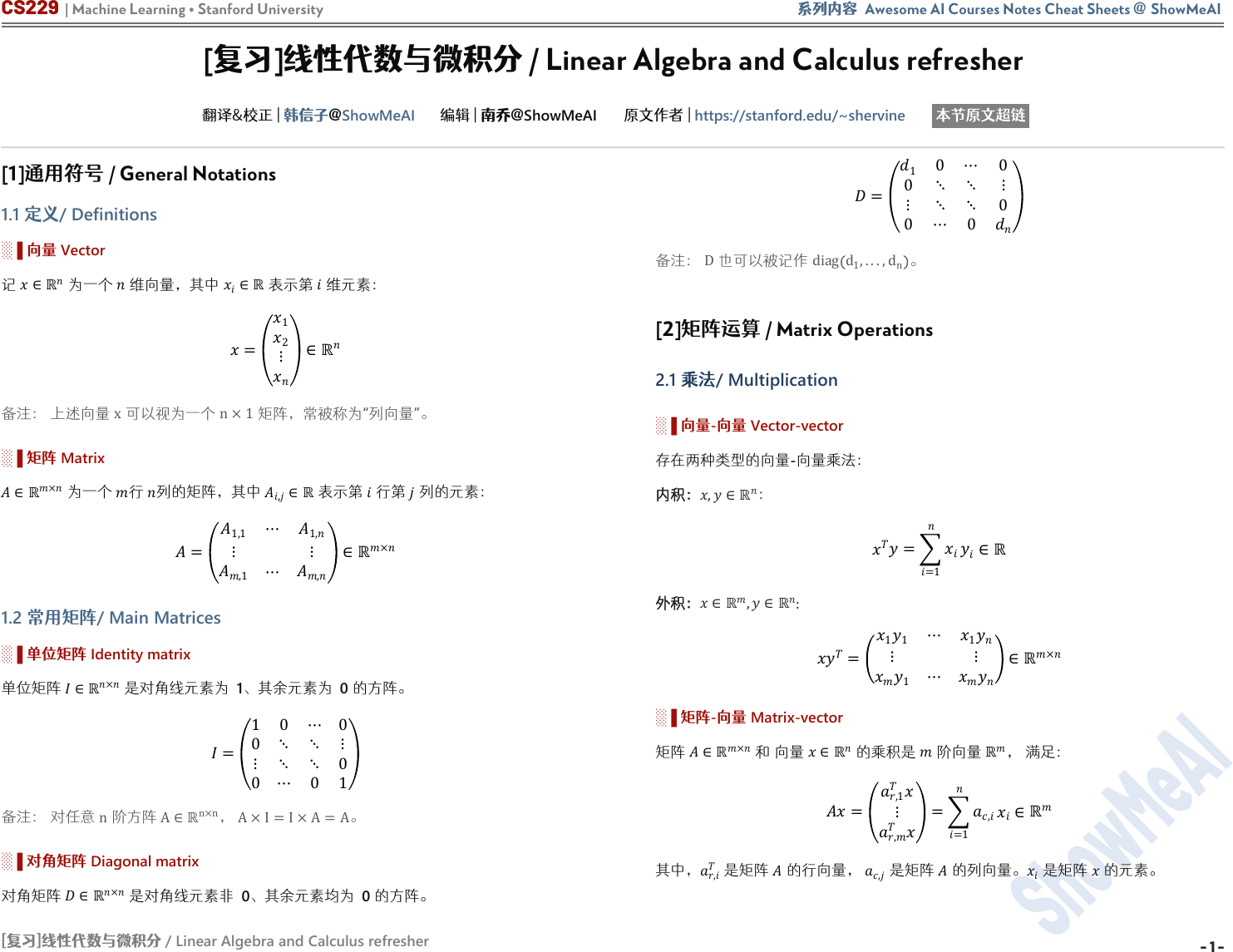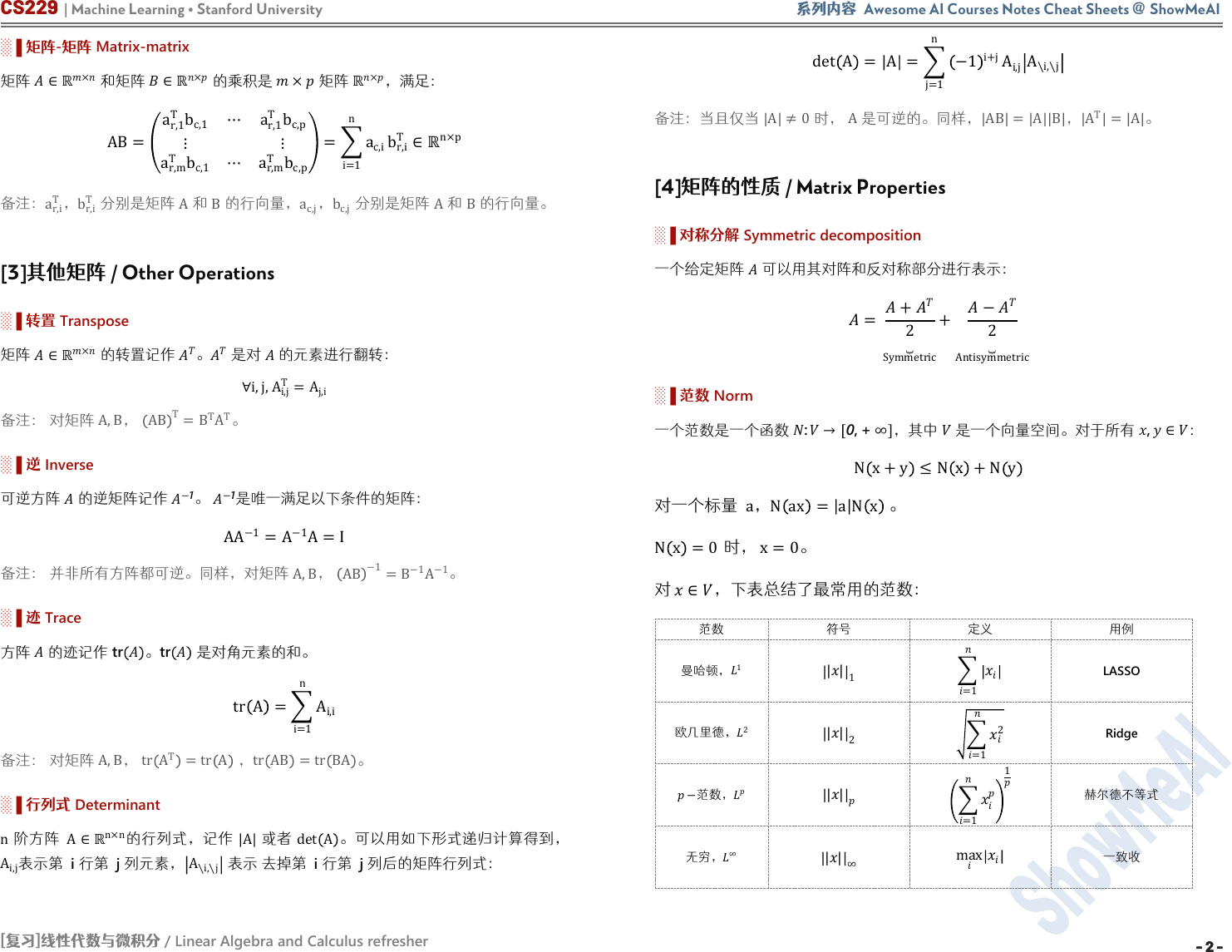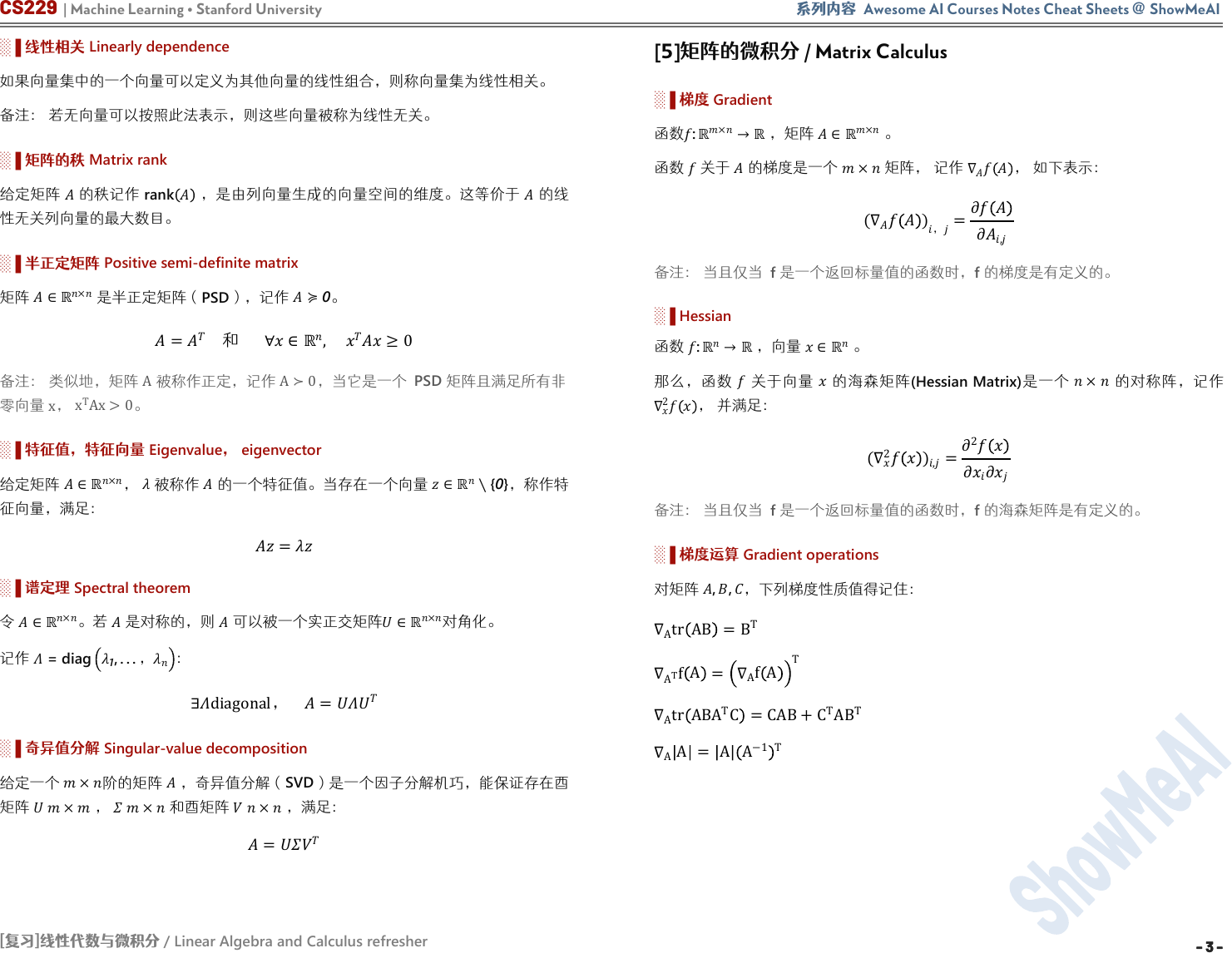CS229 | Machine Learning Stanford University 系列内容 Awesome AI Courses Notes Cheat Sheets @ ShowMeAI
[复习]线性代数与微积分
/ Linear Algebra and Calculus refresher
- 1 -
[

]

/ Linear Algebra and Calculus refresher

ShowMeAI

ShowMeAI

https://stanford.edu/~shervine

通用符号 / General Notations
1.1 定义/ Definitions
░▐ 向量 Vector

=
1
2

x

n×1

░▐ 矩阵
Matrix
×

,

=
1,1
1,
,1
,
×
1.2 常用矩阵/ Main Matrices
░▐ 单位矩阵
Identity matrix

×

1
、其余元素为
0

=
1 0 0
0
0
0 0 1

n

A
n×n
A×I=I×A=A
░▐ 对角矩阵 Diagonal matrix

×

0
、其余元素均为
0

=
1
0 0
0
0
0 0

D

diag d
1
,...,d
n
矩阵运算 / Matrix Operations
2.1 乘法/ Multiplication
░▐ 向量-向量 Vector-vector

,
=
=1

,
:

=
1
1
1
1
×
░▐ 矩阵-向量 Matrix-vector

×

=
,1
,
=
=1
,

,

,CS229 | Machine Learning Stanford University 系列内容 Awesome AI Courses Notes Cheat Sheets @ ShowMeAI
[复习]线性代数与微积分
/ Linear Algebra and Calculus refresher
- 2 -- 2 -
░▐ 矩阵
-

Matrix-matrix

×

×

×

×
，满足：
AB=
a
r,1
T
b
c,1
a
r,1
T
b
c,p
a
r,m
T
b
c,1
a
r,m
T
b
c,p
=
i=1
n
a
c,i
b
r,i
T
n×p

a
r,i
T
b
r,i
T

A
B

a
c,j
b
c,j

A
B

其他矩阵 / Other Operations
░▐ 转置 Transpose

×

∀i,j,A
i,j
T
=A
j,i

A,B
AB
T
=B
T
A
T
░▐ Inverse

1
1

AA
−1
=A
−1
A=I

A,B
AB
−1
=B
−1
A
−1
░▐ Trace

tr
tr

tr
A =
i=1
n
A
i,i

A,B
tr A
T
=tr A
tr AB =tr BA
░▐ 行列式 Determinant
n

A
n×n

A

det A
。可式递得到
A
i,j

A
∖i,∖j

det A = A =
j=1
n
−1
i+j
A
i,j
A
∖i,∖j

A 0

A

AB = A B
A
T
= A
矩阵的性质 / Matrix Properties
░▐ 对称分解 Symmetric decomposition

=
+
2
Symmetric
+
2
Antisymmetric
░▐ 范数
Norm

:
0, +
，其中

,
N
x+y
N x +N y

N x =0

x=0
，下表总结了最常用的范数：

1
||
1
=1
LASSO

2
||
2
=1
2
Ridge

||
=1
1

||
maxCS229 | Machine Learning Stanford University 系列内容 Awesome AI Courses Notes Cheat Sheets @ ShowMeAI
[复习]线性代数与微积分
/ Linear Algebra and Calculus refresher
- 3 -
- 3 -
░▐ 线性相关
Linearly dependence

░▐ 矩阵的秩
Matrix rank

rank
，是由列向量成的向空间的维度。等价于

░▐ 半正定矩阵
Positive semi-definite matrix

×

PSD
），记作
0
=
∀
, 
0

A

A0
，当它是一个
PSD

x
x
T
Ax>0
░▐ 特征值，特征向量 Eigenvalue eigenvector

×

{0}
，称作特

=
░▐ 谱定理 Spectral theorem
×
。若

×

1
, . . .
∃diagonal =
░▐ 奇异值分解
Singular-value decomposition

×

，奇异值分解（
SVD
）是一个因子分解机巧，能保证存在酉

×
×

×
，满足：
=
矩阵的微积分 / Matrix Calculus

:
×
，矩阵
×

×

(∇
)
=
∂
∂
,

░▐
Hessian

:
，向量
(Hessian Matrix)
×
2

(∇
2
)
,
=
2
∂
∂

░▐ 梯度运算

, ,
，下列梯度性质值得记住：
A
tr AB =B
T
A
T
f
A
=
A
f A
T
A
tr ABA
T
C =CAB+C
T
AB
T
A
A = A A
−1 TCS229 | Machine Learning Stanford University 系列内容 Awesome AI Courses Notes Cheat Sheets @ ShowMeAI
[复习]线性代数与微积分
/ Linear Algebra and Calculus refresher
Awesome AI Courses Notes Cheat Sheets
Machine Learning
CS229
Deep Learning
CS230
Natural Language Processing
CS224n
Computer Vision
CS231n
Deep Reinforcement Learning
CS285
Neural Networks for NLP
CS11-747
DL for Self-Driving Cars
6.S094
...
Stanford
Stanford
Stanford
Stanford
UC Berkeley
CMU
MIT
...
ShowMeAI TOP20+ AI 读者

Stanford University Machine Learning CS229 Deep Learning
CS230）课程，是本系列的第一批产出

ShowMeAI

CS229 | Machine Learning @ Stanford University
CS230 | Deep Learning @ Stanford University

Supervised Learning

Unsupervised Learning

Deep Learning

Tips and Tricks

CNN

RNN

Tips and Tricks

Probabilities /Statistics

Linear Algebra and Calculus
GitHub
ShowMeAI
https://github.com/
ShowMeAI-Hub/
ShowMeAI 研究中心